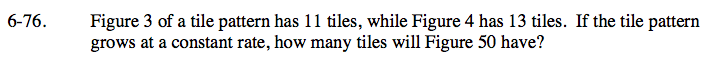### Home > CAAC > Chapter 6 > Lesson 6.2.4 > Problem6-76

6-76.Make either an illustration, a graph, or a table and work backward (using the growth rate) to find the starting number of tiles at Figure 0.

Use the starting number of tiles and the growth rate to write a rule where y = the total number of tiles and x = the figure number.

Plug in 50 for x and solve for y to find the number of tiles that Figure 50 will have.

Figure 50 will have 105 tiles.